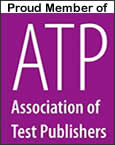2020 Edition

# Electrician Practice Test

Take a free Electrician Practice Test to see what kind of questions are on actual electrician license exams.  Most states require an electrician to pass an exam to receive a journeyman or master electrician license.   State exams cover such areas as Electrical Theory, Trade Knowledge, Grounding and Bonding, Wiring Methods and Installation, Overcurrent Protection, Load Calculations, etc.

To prepare for your actual Electrician Exam, these two practice exams by Ray Holder (Master Electrician and Certified Electrical Trade Instructor) have 300 questions with fully explained answers:

With Tests.com, you have the option to purchase a practice test kit based on the 2011, 2014 or 2017 NEC Codes. Check with your local code official to determine which code applies to your location.

 1. Which of the following is not a type of service disconnect? a. Knife blade switch b. Fuse block c. Circuit Breakers d. Bus Bar
 2. If a grounded conductor is attached to the disconnect switch, which type of service disconnect must be used? a. Circuit breakers b. Knife Blade Switch c. Fuse Block d. None of the above
 3. What is the most frequently used wire size for interior wiring? a. 10 AWG b. 12 AWG c. 15 AWG d. 18 AWG
 4. If you have four to six 12 AWG wires in a conduit, what would be the allowable current-carrying capacity in percentage to the normal? a. 50% b. 60% c. 70% d. 80%
 5. If a box is to contain four No. 10 conductors and two No. 12 conductors, what is the minimum sized box that can be used in cubic inches? a. 12 b. 13 1/2 c. 14 1/2 d. 15
 6. A run of electrical conduit may not have more than _____________ quarter bends between fittings and outlets? a. 2 b. 3 c. 4 d. 5
 7. The total load on any single overcurrent device in a panelboard must not exceed 80 percent of its capacity where the load continues for 3 or more hours to avoid overheating of the conductor. True False
 8. A fuse should be rated higher than the lowest rated component in a panelboard to be protected. True False
 9. Which of the following locations would most likely require installation of a ground fault circuit interrupter? a. living room b. closet c. bedroom d. bathroom
 10. An open circuit occurs in a wiring system when _____________________. a. the system is working properly b. a conductor is broken or separated c. a fuse is installed d. None of the above
 11. Short circuits or grounds can be all of the following except __________________. a. Solid b. Partial c. Floating d. Contracted
 12. Which phase is probably open when you test Phase A and Phase B and get a low voltage reading, test Phase B and C and get a normal reading and test Phase A and C and get a low voltage reading? a. Phase A b. Phase B c. Phase C d. None of the above
 13. In residential buildings, small appliance loads should have two or more ________________. a. 15 ampere branch circuits b. 20 ampere branch circuits c. 40 ampere branch circuits d. 50 ampere branch circuits
 14. What law would you use to determine the voltage of a power supply? a. Coulomb's Law b. Kirchoff's Law c. Ohm's Law d. None of the above
 15. Ohm's law states ____________________. a. Voltage = amps x resistance b. Voltage = current / resistance c. Voltage = watts x current d. Voltage = current/watts e. None of the above
 16. The amount that a given conductor resists the flow of electric current is measured in___________. a. Watts b. Volts c. Iambs d. Coulombs e. Ohms
 17. Which law holds that like-charged objects repel and opposite- charged objects attract? a. Coulomb's Law b. Kirchoff's Law c. Ohm's Law d. None of the above
 18. Direct current can change direction. True False
 19. Alternating current changes direction at which of the following frequencies? a. 40 Hz b. 50 Hz c. 60 Hz d. 70 Hz e. None of the above
 20. Electron Theory and Conventional Theory hold opposite current flow directions. True False
 21. Which of the following will not affect the resistance of a circuit? a. Length of the Conductor b. Diameter of the Conductor c. Insulation of the Conductor d. Temperature e. Physical Condition
 22. Which of the following are a source of electricity? a. Friction b. Heat c. Light d. Pressure e. Chemical action f. All of the above
 23. In a series circuit, the current has ___________ path(s) to take. a. 1 b. 2 c. 3 d. 4 e. 5
 24. When connecting a voltmeter lnto a DC circuit, what should be observed? a. Power Factor b. Rms c. Resistance d. Polarity

Quality starts with who wrote the material.
Our practice exam writer
s
:
Ray Holder